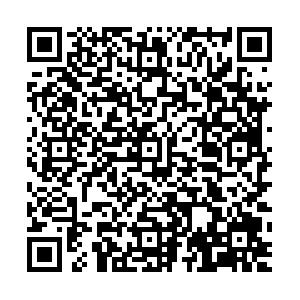• ISSN 1006-3080
• CN 31-1691/TQ

 引用本文: 胡剑峰, 周家乐, 王慧锋, 张俊. 一种电熔焊接缺陷自动识别方法的研究与应用[J]. 华东理工大学学报（自然科学版）.HU Jianfeng, ZHOU Jiale, WANG Huifeng, ZHANG Jun. Research and Application of An Automatic Identification Method for Electrofusion Welding Defects[J]. Journal of East China University of Science and Technology. doi: 10.14135/j.cnki.1006-3080.20210316001
 Citation: HU Jianfeng, ZHOU Jiale, WANG Huifeng, ZHANG Jun. Research and Application of An Automatic Identification Method for Electrofusion Welding Defects[J]. Journal of East China University of Science and Technology.• 中图分类号: TE88

## Research and Application of An Automatic Identification Method for Electrofusion Welding Defects

• 摘要: 聚乙烯（Polyethylene，PE）燃气管道的电熔焊接状况可通过相控阵系统拍摄的超声波图像获得，但是否存在焊接缺陷目前都是由专业人员人工识别每张图片中的特征线、电阻丝、底面回波线等与缺陷相关的信息，进而确定缺陷类别和等级，这种方法费时、费力且容易发生漏检和错检现象。本文针对PE管道电熔焊接缺陷的识别问题提出了一种基于图像处理技术的焊接缺陷自动识别方法，同时判断超声图像中存在缺陷的类别和等级。该方法由4个步骤组成：（1）通过数据增强技术扩充已有图片数量，构建数据集；（2）训练图像语义分割模型，对图像进行语义分割；（3）采用数学形态学方法去除分割结果的噪声，并通过连通域分析法得到缺陷相关信息；（4）依据焊接标准及缺陷相关信息识别缺陷类别及等级。实验结果表明，本文提出的缺陷识别方法在准确率、召回率以及运行时间方面均达到了工业应用要求。

• 图  1  超声图像

Figure  1.  Ultrasonic image

图  2  Res-block结构图

Figure  2.  Structure of Res-block

图  3  残差U-Net网络结构

Figure  3.  Structure of residual U-Net network

图  4  电熔焊接数据增强

Figure  4.  Data enhancement of electrofusion welding

图  5  焊接标注图

Figure  5.  Labeling image of welding

图  6  分割模型效果对比

Figure  6.  Effect comparison of segmentation model

图  7  噪声图

Figure  7.  Image with noise

图  8  去噪图

Figure  8.  Denoising image

图  9  连通域标记

Figure  9.  Connected domain marking

表  1  标准相关的几何参数

Table  1.   Geometric parameters related to the standard

 Classes Geometric parameters Meaning of parameters Feature line $f_{x\,\rm{cen} }\left( k \right)$ The x-coordinate of the centroid of the kth connected domain of the feature line category $f_{y\,\rm{cen} }\left( k \right)$ The y-coordinate of the centroid of the kth connected domain of the feature line category Resistance wire $r_{x\,\rm{cen} }\left( n \right)$ The x-coordinate of the centroid of the nth connected domain of the resistance wire category $r_{y\,\rm{cen} }\left( n \right)$ The y-coordinate of the centroid of the nth connected domain of the resistance wire category $r_{x\min }\left( n \right)$ The minimum value of the nth connected domain of the resistance wire category in the x-axis $r_{x\max} \left( n \right)$ The maximum value of the nth connected domain of the resistance wire category in the x-axis Bottom $b_{x\min}\left( m \right)$ The minimum value of the mth connected domain of the bottom category in the x-axis $b_{x\max}\left( m \right)$ The maximum value of the mth connected domain of the bottom category in the x-axis

表  2  冷/过焊缺陷表征程度与缺陷类型、等级对照

Table  2.   Comparison of cold/over-welding defect characterization degree, defect categories and levels

 Characterization degree /% Defect categories /level $-100\leqslant H_{\rm{cold/over}}\leqslant -40$ Over welding/Ⅲ $-40 < H_{\rm{cold/over}}\leqslant -20$ Over welding/Ⅱ $-20 < H_{\rm{cold/over}} < 0$ Over welding/Ⅰ $H_{\rm{cold/over}}=0$ Normal $0 < H_{\rm{cold/over}} < 10$ Cold welding/Ⅰ $10\leqslant H_{\rm{cold/over}} < 30$ Cold welding/Ⅱ $30\leqslant H_{\rm{cold/over}}\leqslant 100$ Cold welding/Ⅲ

表  3  孔洞缺陷表征程度与缺陷类型、等级对照

Table  3.   Comparison of hole defect characterization degree, defect categories and levels

 Number of connected domains Characterization degree/% Defect categories/levels M=2 $0 < H_{\rm{hole}} < 5$ Single hole/Ⅰ $5\leqslant H_{\rm{hole}} < 10$ Single hole/Ⅱ $H_{\rm{hole}}\geqslant 10$ Single hole/Ⅲ ${ M} > 2$ $0 < H_{\rm{hole}} < 10$ Multiple holes/Ⅰ $10\leqslant H_{\rm{hole}} < 15$ Multiple holes/Ⅱ $H_{\rm{hole}}\geqslant 15$ Multiple holes/Ⅲ

表  4  电阻丝连通域最大偏离量与缺陷等级对照

Table  4.   Comparison between the maximum deviation of the connected domain of the resistance wire and defect levels

 $d_{\max}$ Defect levels $d_{\max} < \dfrac{1}{2}x'$ Ⅰ $\dfrac{1}{2}x'\leqslant d_{\max} < x'$ Ⅱ $d_{\max}\geqslant x'$ Ⅲ

表  5  二分类结果

Table  5.   Results of binary classification

 Accuracy/% Recall/% 92.8 95.5

表  6  多类别识别结果

Table  6.   Test results of multiple categories

 Defect category Recall/% Cold welding 96.7 Over welding 96.7 Misalignment 93.8 Inclusion 100.0 Hole 100.0

表  7  冷/过焊缺陷等级划分结果

Table  7.   Results of classification of cold/over welding defects

 Levels Recall(Cold welding)/% Recall(Over welding)/% Ⅰ 80.0 83.3 Ⅱ 90.0 80.0 Ⅲ 93.3 94.7
•点击查看大图
##### 计量
• 文章访问数:  277
• HTML全文浏览量:  152
• PDF下载量:  36
• 被引次数: 0
##### 出版历程
• 收稿日期:  2021-03-16
• 网络出版日期:  2021-07-01

### 目录/下载:  全尺寸图片 幻灯片
• 分享
• 用微信扫码二维码

分享至好友和朋友圈